Choose Classifier Options

Choose Classifier Type

You can use Classification Learner to automatically train a selection of different classification models on your data. Use automated training to quickly try a selection of model types, then explore promising models interactively. To get started, try these options first: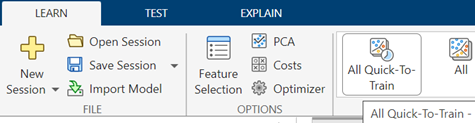Get Started Classifier ButtonsDescription
All Quick-To-TrainTry this first. The app will train all the model types available for your data set that are typically fast to fit.
All LinearTry this if you expect linear boundaries between the classes in your data. This option fits only Linear SVM and Linear Discriminant.
AllUse this to train all available nonoptimizable model types. Trains every type regardless of any prior trained models. Can be time-consuming.

If you want to explore classifiers one at a time, or you already know what classifier type you want, you can select individual models or train a group of the same type. To see all available classifier options, on the Classification Learner tab, click the arrow in the Model Type section to expand the list of classifiers. The nonoptimizable model options in the Model Type gallery are preset starting points with different settings, suitable for a range of different classification problems. To use optimizable model options and tune model hyperparameters automatically, see Hyperparameter Optimization in Classification Learner App.

For help choosing the best classifier type for your problem, see the table showing typical characteristics of different supervised learning algorithms. Use the table as a guide for your final choice of algorithms. Decide on the tradeoff you want in speed, flexibility, and interpretability. The best classifier type depends on your data.

Tip

To avoid overfitting, look for a model of lower flexibility that provides sufficient accuracy. For example, look for simple models such as decision trees and discriminants that are fast and easy to interpret. If the models are not accurate enough predicting the response, choose other classifiers with higher flexibility, such as ensembles. To control flexibility, see the details for each classifier type.

Characteristics of Classifier Types

ClassifierInterpretability
Decision TreesEasy
Discriminant AnalysisEasy
Logistic RegressionEasy
Naive Bayes ClassifiersEasy

Support Vector MachinesEasy for Linear SVM.
Hard for all other kernel types.
Nearest Neighbor ClassifiersHard
Kernel Approximation ClassifiersHard
Ensemble ClassifiersHard
Neural Network ClassifiersHard

To read a description of each classifier in Classification Learner, switch to the details view.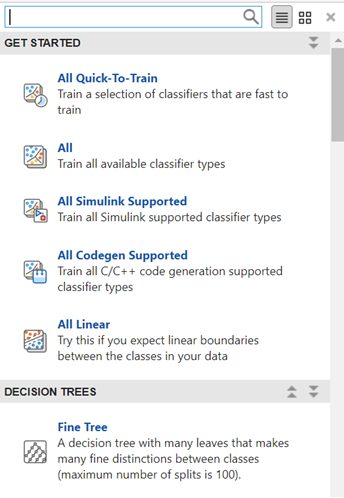Tip

After you choose a classifier type (for example, decision trees), try training using each of the classifiers. The nonoptimizable options in the Model Type gallery are starting points with different settings. Try them all to see which option produces the best model with your data.

For workflow instructions, see Train Classification Models in Classification Learner App.

Categorical Predictor Support

In Classification Learner, the Model Type gallery shows as available the classifier types that support your selected data.

ClassifierAll predictors numericAll predictors categoricalSome categorical, some numeric
Decision TreesYesYesYes
Discriminant AnalysisYesNoNo
Logistic RegressionYesYesYes
Naive BayesYesYesYes
SVMYesYesYes
Nearest NeighborEuclidean distance onlyHamming distance onlyNo
Kernel ApproximationYesYesYes
EnsemblesYesYes, except Subspace DiscriminantYes, except any Subspace
Neural NetworksYesYesYes

Decision Trees

Decision trees are easy to interpret, fast for fitting and prediction, and low on memory usage, but they can have low predictive accuracy. Try to grow simpler trees to prevent overfitting. Control the depth with the Maximum number of splits setting.

Tip

Model flexibility increases with the Maximum number of splits setting.

Classifier TypeInterpretabilityModel Flexibility
Coarse TreeEasyLow
Few leaves to make coarse distinctions between classes (maximum number of splits is 4).
Medium TreeEasyMedium
Medium number of leaves for finer distinctions between classes (maximum number of splits is 20).
Fine TreeEasyHigh
Many leaves to make many fine distinctions between classes (maximum number of splits is 100).

Tip

In the Model Type gallery click to try each of the nonoptimizable decision tree options. Train them all to see which settings produce the best model with your data. Select the best model in the Models pane. To try to improve your model, try feature selection, and then try changing some advanced options.

You train classification trees to predict responses to data. To predict a response, follow the decisions in the tree from the root (beginning) node down to a leaf node. The leaf node contains the response. Statistics and Machine Learning Toolbox™ trees are binary. Each step in a prediction involves checking the value of one predictor (variable). For example, here is a simple classification tree:This tree predicts classifications based on two predictors, x1 and x2. To predict, start at the top node. At each decision, check the values of the predictors to decide which branch to follow. When the branches reach a leaf node, the data is classified either as type 0 or 1.

You can visualize your decision tree model by exporting the model from the app, and then entering:

view(trainedModel.ClassificationTree,'Mode','graph')
The figure shows an example fine tree trained with the fisheriris data.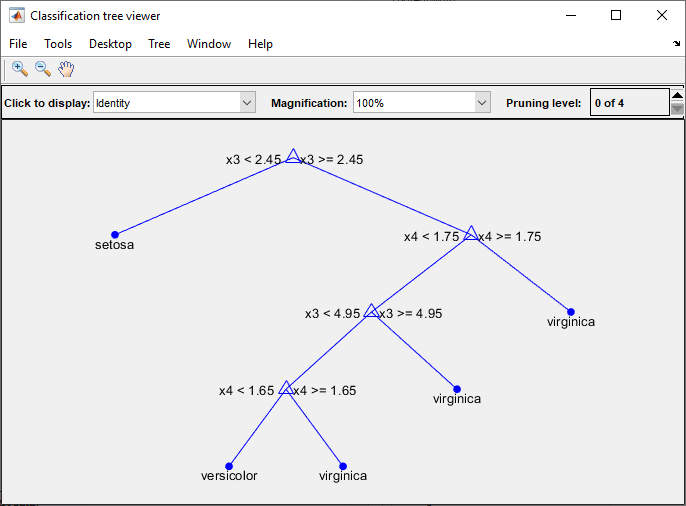Classification trees in Classification Learner use the fitctree function. You can set these options:

• Maximum number of splits

Specify the maximum number of splits or branch points to control the depth of your tree. When you grow a decision tree, consider its simplicity and predictive power. To change the number of splits, click the buttons or enter a positive integer value in the Maximum number of splits box.

• A fine tree with many leaves is usually highly accurate on the training data. However, the tree might not show comparable accuracy on an independent test set. A leafy tree tends to overtrain, and its validation accuracy is often far lower than its training (or resubstitution) accuracy.

• In contrast, a coarse tree does not attain high training accuracy. But a coarse tree can be more robust in that its training accuracy can approach that of a representative test set. Also, a coarse tree is easy to interpret.

• Split criterion

Specify the split criterion measure for deciding when to split nodes. Try each of the three settings to see if they improve the model with your data.

Split criterion options are Gini's diversity index, Twoing rule, or Maximum deviance reduction (also known as cross entropy).

The classification tree tries to optimize to pure nodes containing only one class. Gini's diversity index (the default) and the deviance criterion measure node impurity. The twoing rule is a different measure for deciding how to split a node, where maximizing the twoing rule expression increases node purity.

For details of these split criteria, see ClassificationTree More About.

• Surrogate decision splits — Only for missing data.

Specify surrogate use for decision splits. If you have data with missing values, use surrogate splits to improve the accuracy of predictions.

When you set Surrogate decision splits to On, the classification tree finds at most 10 surrogate splits at each branch node. To change the number, click the buttons or enter a positive integer value in the Maximum surrogates per node box.

When you set Surrogate decision splits to Find All, the classification tree finds all surrogate splits at each branch node. The Find All setting can use considerable time and memory.

Alternatively, you can let the app choose some of these model options automatically by using hyperparameter optimization. See Hyperparameter Optimization in Classification Learner App.

Discriminant Analysis

Discriminant analysis is a popular first classification algorithm to try because it is fast, accurate and easy to interpret. Discriminant analysis is good for wide datasets.

Discriminant analysis assumes that different classes generate data based on different Gaussian distributions. To train a classifier, the fitting function estimates the parameters of a Gaussian distribution for each class.

Classifier TypeInterpretabilityModel Flexibility
Linear DiscriminantEasyLow
Creates linear boundaries between classes.EasyLow
Creates nonlinear boundaries between classes (ellipse, parabola or hyperbola).

Discriminant analysis in Classification Learner uses the fitcdiscr function. For both linear and quadratic discriminants, you can change the Covariance structure option. If you have predictors with zero variance or if any of the covariance matrices of your predictors are singular, training can fail using the default, Full covariance structure. If training fails, select the Diagonal covariance structure instead.

Alternatively, you can let the app choose some of these model options automatically by using hyperparameter optimization. See Hyperparameter Optimization in Classification Learner App.

Logistic Regression

If you have 2 classes, logistic regression is a popular simple classification algorithm to try because it is easy to interpret. The classifier models the class probabilities as a function of the linear combination of predictors.

Classifier TypeInterpretabilityModel Flexibility
Logistic RegressionEasyLow
You cannot change any parameters to control model flexibility.

Logistic regression in Classification Learner uses the fitglm function. You cannot set any options for this classifier in the app.

Naive Bayes Classifiers

Naive Bayes classifiers are easy to interpret and useful for multiclass classification. The naive Bayes algorithm leverages Bayes theorem and makes the assumption that predictors are conditionally independent, given the class. Use these classifiers if this independence assumption is valid for predictors in your data. However, the algorithm still appears to work well when the independence assumption is not valid.

For kernel naive Bayes classifiers, you can control the kernel smoother type with the Kernel Type setting, and control the kernel smoothing density support with the Support setting.

Classifier TypeInterpretabilityModel Flexibility
Gaussian Naive BayesEasyLow
You cannot change any parameters to control model flexibility.
Kernel Naive BayesEasyMedium
You can change settings for Kernel Type and Support to control how the classifier models predictor distributions.

Naive Bayes in Classification Learner uses the fitcnb function.

For kernel naive Bayes classifiers, you can set these options:

• Kernel Type — Specify the kernel smoother type. Try setting each of these options to see if they improve the model with your data.

Kernel type options are Gaussian, Box, Epanechnikov, or Triangle.

• Support — Specify the kernel smoothing density support. Try setting each of these options to see if they improve the model with your data.

Support options are Unbounded (all real values) or Positive (all positive real values).

Alternatively, you can let the app choose some of these model options automatically by using hyperparameter optimization. See Hyperparameter Optimization in Classification Learner App.

For next steps training models, see Train Classification Models in Classification Learner App.

Support Vector Machines

In Classification Learner, you can train SVMs when your data has two or more classes.

Classifier TypeInterpretabilityModel Flexibility
Linear SVMEasyLow
Makes a simple linear separation between classes.HardMedium
Cubic SVMHardMedium
Fine Gaussian SVMHardHigh — decreases with kernel scale setting.
Makes finely detailed distinctions between classes, with kernel scale set to sqrt(P)/4.
Medium Gaussian SVMHardMedium
Medium distinctions, with kernel scale set to sqrt(P).
Coarse Gaussian SVMHardLow
Makes coarse distinctions between classes, with kernel scale set to sqrt(P)*4, where P is the number of predictors.

Tip

Try training each of the nonoptimizable support vector machine options in the Model Type gallery. Train them all to see which settings produce the best model with your data. Select the best model in the Models pane. To try to improve your model, try feature selection, and then try changing some advanced options.

An SVM classifies data by finding the best hyperplane that separates data points of one class from those of the other class. The best hyperplane for an SVM means the one with the largest margin between the two classes. Margin means the maximal width of the slab parallel to the hyperplane that has no interior data points.

The support vectors are the data points that are closest to the separating hyperplane; these points are on the boundary of the slab. The following figure illustrates these definitions, with + indicating data points of type 1, and – indicating data points of type –1.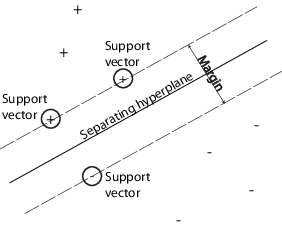SVMs can also use a soft margin, meaning a hyperplane that separates many, but not all data points.

For an example, see Train Support Vector Machines Using Classification Learner App.

If you have exactly two classes, Classification Learner uses the fitcsvm function to train the classifier. If you have more than two classes, the app uses the fitcecoc function to reduce the multiclass classification problem to a set of binary classification subproblems, with one SVM learner for each subproblem. To examine the code for the binary and multiclass classifier types, you can generate code from your trained classifiers in the app.

You can set these options in the app:

• Kernel function

Specify the Kernel function to compute the classifier.

• Linear kernel, easiest to interpret

• Gaussian or Radial Basis Function (RBF) kernel

• Cubic

• Box constraint level

Specify the box constraint to keep the allowable values of the Lagrange multipliers in a box, a bounded region.

To tune your SVM classifier, try increasing the box constraint level. Click the buttons or enter a positive scalar value in the Box constraint level box. Increasing the box constraint level can decrease the number of support vectors, but also can increase training time.

The Box Constraint parameter is the soft-margin penalty known as C in the primal equations, and is a hard “box” constraint in the dual equations.

• Kernel scale mode

Specify manual kernel scaling if desired.

When you set Kernel scale mode to Auto, then the software uses a heuristic procedure to select the scale value. The heuristic procedure uses subsampling. Therefore, to reproduce results, set a random number seed using rng before training the classifier.

When you set Kernel scale mode to Manual, you can specify a value. Click the buttons or enter a positive scalar value in the Manual kernel scale box. The software divides all elements of the predictor matrix by the value of the kernel scale. Then, the software applies the appropriate kernel norm to compute the Gram matrix.

Tip

Model flexibility decreases with the kernel scale setting.

• Multiclass method

Only for data with 3 or more classes. This method reduces the multiclass classification problem to a set of binary classification subproblems, with one SVM learner for each subproblem. One-vs-One trains one learner for each pair of classes. It learns to distinguish one class from the other. One-vs-All trains one learner for each class. It learns to distinguish one class from all others.

• Standardize data

Specify whether to scale each coordinate distance. If predictors have widely different scales, standardizing can improve the fit.

Alternatively, you can let the app choose some of these model options automatically by using hyperparameter optimization. See Hyperparameter Optimization in Classification Learner App.

Nearest Neighbor Classifiers

Nearest neighbor classifiers typically have good predictive accuracy in low dimensions, but might not in high dimensions. They have high memory usage, and are not easy to interpret.

Tip

Model flexibility decreases with the Number of neighbors setting.

Classifier TypeInterpretabilityModel Flexibility
Fine KNNHard

Finely detailed distinctions between classes. The number of neighbors is set to 1.

Medium KNNHard

Medium distinctions between classes. The number of neighbors is set to 10.

Coarse KNNHard

Coarse distinctions between classes. The number of neighbors is set to 100.

Cosine KNNHardMedium distinctions between classes, using a Cosine distance metric. The number of neighbors is set to 10.
Cubic KNNHardMedium distinctions between classes, using a cubic distance metric. The number of neighbors is set to 10.
Weighted KNNHardMedium distinctions between classes, using a distance weight. The number of neighbors is set to 10.

Tip

Try training each of the nonoptimizable nearest neighbor options in the Model Type gallery. Train them all to see which settings produce the best model with your data. Select the best model in the Models pane. To try to improve your model, try feature selection, and then (optionally) try changing some advanced options.

What is k-Nearest Neighbor classification? Categorizing query points based on their distance to points (or neighbors) in a training dataset can be a simple yet effective way of classifying new points. You can use various metrics to determine the distance. Given a set X of n points and a distance function, k-nearest neighbor (kNN) search lets you find the k closest points in X to a query point or set of points. kNN-based algorithms are widely used as benchmark machine learning rules.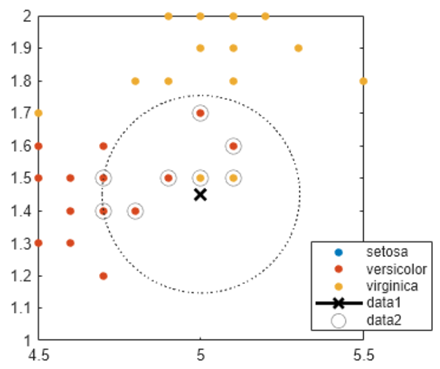For an example, see Train Nearest Neighbor Classifiers Using Classification Learner App.

Nearest Neighbor classifiers in Classification Learner use the fitcknn function. You can set these options:

• Number of neighbors

Specify the number of nearest neighbors to find for classifying each point when predicting. Specify a fine (low number) or coarse classifier (high number) by changing the number of neighbors. For example, a fine KNN uses one neighbor, and a coarse KNN uses 100. Many neighbors can be time consuming to fit.

• Distance metric

You can use various metrics to determine the distance to points. For definitions, see the class ClassificationKNN.

• Distance weight

Specify the distance weighting function. You can choose Equal (no weights), Inverse (weight is 1/distance), or Squared Inverse (weight is 1/distance2).

• Standardize data

Specify whether to scale each coordinate distance. If predictors have widely different scales, standardizing can improve the fit.

Alternatively, you can let the app choose some of these model options automatically by using hyperparameter optimization. See Hyperparameter Optimization in Classification Learner App.

Kernel Approximation Classifiers

In Classification Learner, you can use kernel approximation classifiers to perform nonlinear classification of data with many observations. For large in-memory data, kernel classifiers tend to train and predict faster than SVM classifiers with Gaussian kernels.

The Gaussian kernel classification models map predictors in a low-dimensional space into a high-dimensional space, and then fit a linear model to the transformed predictors in the high-dimensional space. Choose between fitting an SVM linear model and fitting a logistic regression linear model in the expanded space.

Tip

In the Model Type gallery, click All Kernelsto try each of the preset kernel approximation options and see which settings produce the best model with your data. Select the best model in the Models pane, and try to improve that model by using feature selection and changing some advanced options.

Classifier TypeInterpretabilityModel Flexibility
SVM KernelHardMedium — increases as the Kernel scale setting decreases
Logistic Regression KernelHardMedium — increases as the Kernel scale setting decreases

For an example, see Train Kernel Approximation Classifiers Using Classification Learner App.

If you have exactly two classes, Classification Learner uses the fitckernel function to train kernel classifiers. If you have more than two classes, the app uses the fitcecoc function to reduce the multiclass classification problem to a set of binary classification subproblems, with one kernel learner for each subproblem.

You can set these options:

• Learner — Specify the linear classification model type to fit in the expanded space, either SVM or Logistic Regression. SVM kernel classifiers use a hinge loss function during model fitting, whereas logistic regression kernel classifiers use a deviance (logistic) loss.

• Number of expansion dimensions — Specify the number of dimensions in the expanded space.

• When you set this option to Auto, the software sets the number of dimensions to 2.^ceil(min(log2(p)+5,15)), where p is the number of predictors.

• When you set this option to Manual, you can specify a value by clicking the buttons or entering a positive scalar value in the box.

• Regularization strength (Lambda) — Specify the ridge (L2) regularization penalty term. When you use an SVM learner, the box constraint C and the regularization term strength λ are related by C = 1/(λn), where n is the number of observations.

• When you set this option to Auto, the software sets the regularization strength to 1/n, where n is the number of observations.

• When you set this option to Manual, you can specify a value by clicking the buttons or entering a positive scalar value in the box.

• Kernel scale — Specify the kernel scaling. The software uses this value to obtain a random basis for the random feature expansion. For more details, see Random Feature Expansion.

• When you set this option to Auto, the software uses a heuristic procedure to select the scale value. The heuristic procedure uses subsampling. Therefore, to reproduce results, set a random number seed using rng before training the classifier.

• When you set this option to Manual, you can specify a value by clicking the buttons or entering a positive scalar value in the box.

• Multiclass method — Specify the method for reducing the multiclass problem to a set of binary subproblems, with one kernel learner for each subproblem. This value is applicable only for data with more than two classes.

• One-vs-One trains one learner for each pair of classes. This method learns to distinguish one class from the other.

• One-vs-All trains one learner for each class. This method learns to distinguish one class from all others.

• Iteration limit — Specify the maximum number of training iterations.

Ensemble Classifiers

Ensemble classifiers meld results from many weak learners into one high-quality ensemble model. Qualities depend on the choice of algorithm.

Tip

Model flexibility increases with the Number of learners setting.

All ensemble classifiers tend to be slow to fit because they often need many learners.

Classifier TypeInterpretabilityEnsemble MethodModel Flexibility
Boosted TreesMedium to high — increases with Number of learners or Maximum number of splits setting.

Tip

Boosted trees can usually do better than bagged, but might require parameter tuning and more learners

Bagged TreesHardRandom forest
Bag, with Decision Tree learners

High — increases with Number of learners setting.

Tip

Try this classifier first.

Subspace DiscriminantHardSubspace, with Discriminant learners

Medium — increases with Number of learners setting.

Good for many predictors

Subspace KNNHardSubspace, with Nearest Neighbor learners

Medium — increases with Number of learners setting.

Good for many predictors

RUSBoost TreesHardRUSBoost, with Decision Tree learners

Medium — increases with Number of learners or Maximum number of splits setting.

Good for skewed data (with many more observations of 1 class)

GentleBoost or LogitBoost — not available in the Model Type gallery.
If you have 2 class data, select manually.
HardGentleBoost or LogitBoost, with Decision Tree learners
Choose Boosted Trees and change to GentleBoost method.

Medium — increases with Number of learners or Maximum number of splits setting.

For binary classification only

Bagged trees use Breiman's 'random forest' algorithm. For reference, see Breiman, L. Random Forests. Machine Learning 45, pp. 5–32, 2001.

Tips

• Try bagged trees first. Boosted trees can usually do better but might require searching many parameter values, which is time-consuming.

• Try training each of the nonoptimizable ensemble classifier options in the Model Type gallery. Train them all to see which settings produce the best model with your data. Select the best model in the Models pane. To try to improve your model, try feature selection, PCA, and then (optionally) try changing some advanced options.

• For boosting ensemble methods, you can get fine detail with either deeper trees or larger numbers of shallow trees. As with single tree classifiers, deep trees can cause overfitting. You need to experiment to choose the best tree depth for the trees in the ensemble, in order to trade-off data fit with tree complexity. Use the Number of learners and Maximum number of splits settings.

For an example, see Train Ensemble Classifiers Using Classification Learner App.

Ensemble classifiers in Classification Learner use the fitcensemble function. You can set these options:

• For help choosing Ensemble method and Learner type, see the Ensemble table. Try the presets first.

• Maximum number of splits

For boosting ensemble methods, specify the maximum number of splits or branch points to control the depth of your tree learners. Many branches tend to overfit, and simpler trees can be more robust and easy to interpret. Experiment to choose the best tree depth for the trees in the ensemble.

• Number of learners

Try changing the number of learners to see if you can improve the model. Many learners can produce high accuracy, but can be time consuming to fit. Start with a few dozen learners, and then inspect the performance. An ensemble with good predictive power can need a few hundred learners.

• Learning rate

Specify the learning rate for shrinkage. If you set the learning rate to less than 1, the ensemble requires more learning iterations but often achieves better accuracy. 0.1 is a popular choice.

• Subspace dimension

For subspace ensembles, specify the number of predictors to sample in each learner. The app chooses a random subset of the predictors for each learner. The subsets chosen by different learners are independent.

Alternatively, you can let the app choose some of these model options automatically by using hyperparameter optimization. See Hyperparameter Optimization in Classification Learner App.

Neural Network Classifiers

Neural network models typically have good predictive accuracy and can be used for multiclass classification; however, they are not easy to interpret.

Model flexibility increases with the size and number of fully connected layers in the neural network.

Tip

In the Model Type gallery, click All Neural Networksto try each of the preset neural network options and see which settings produce the best model with your data. Select the best model in the Models pane, and try to improve that model by using feature selection and changing some advanced options.

Classifier TypeInterpretabilityModel Flexibility
Narrow Neural NetworkHardMedium — increases with the First layer size setting
Medium Neural NetworkHardMedium — increases with the First layer size setting
Wide Neural NetworkHardMedium — increases with the First layer size setting
Bilayered Neural NetworkHardHigh — increases with the First layer size and Second layer size settings
Trilayered Neural NetworkHardHigh — increases with the First layer size, Second layer size, and Third layer size settings

Each model is a feedforward, fully connected neural network for classification. The first fully connected layer of the neural network has a connection from the network input (predictor data), and each subsequent layer has a connection from the previous layer. Each fully connected layer multiplies the input by a weight matrix and then adds a bias vector. An activation function follows each fully connected layer. The final fully connected layer and the subsequent softmax activation function produce the network's output, namely classification scores (posterior probabilities) and predicted labels. For more information, see Neural Network Structure.

For an example, see Train Neural Network Classifiers Using Classification Learner App.

Neural network classifiers in Classification Learner use the fitcnet function. You can set these options:

• Number of fully connected layers — Specify the number of fully connected layers in the neural network, excluding the final fully connected layer for classification. You can choose a maximum of three fully connected layers.

• First layer size, Second layer size, and Third layer size — Specify the size of each fully connected layer, excluding the final fully connected layer. If you choose to create a neural network with multiple fully connected layers, consider specifying layers with decreasing sizes.

• Activation — Specify the activation function for all fully connected layers, excluding the final fully connected layer. The activation function for the last fully connected layer is always softmax. Choose from the following activation functions: ReLU, Tanh, None, and Sigmoid.

• Iteration limit — Specify the maximum number of training iterations.

• Regularization strength (Lambda) — Specify the ridge (L2) regularization penalty term.

• Standardize data — Specify whether to standardize the numeric predictors. If predictors have widely different scales, standardizing can improve the fit. Standardizing the data is highly recommended.

Alternatively, you can let the app choose some of these model options automatically by using hyperparameter optimization. See Hyperparameter Optimization in Classification Learner App.